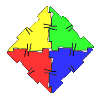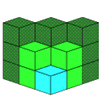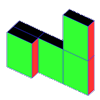#### You may also like### Polydron

This activity investigates how you might make squares and pentominoes from Polydron.If you had 36 cubes, what different cuboids could you make?### Cereal Packets

How can you put five cereal packets together to make different shapes if you must put them face-to-face?

# Cat Food

##### Age 7 to 11 Challenge Level:

Many people worked on this problem, sent us splendid 'write-ups' and invented new problems of this type which will appear in the following months. Some people made a table of the triangle numbers and some made use of algebra. Congratulations to Kerry and Kayleigh from Lasswade High School; to Lyndsay, Sheila, Helen, Suzanne, Peach, Cheryl, Peggy, Jennifer, Pen, Laura and Emma from The Mount School York; to Ian from Cooper's Coburn School; to Hannah from Stamford High School; and to Natalia, Caroline, James and Katherine, from Hethersett High School, Norfolk.

Q: How high would the stack be? Would it be taller than you are?

To answer this I measured the height of a few baked beans and spaghetti tins at home and found that the average height was 11 cm. I then did the sum

$11 \times 14 = 154$.

So the height of the stack would be about 154 cm. My own height is 1 m 73 cm and the difference is 19 cm, so I would be taller than the stack. (James)

I am 149 cm so they are taller than me. (Kerry)

It wouldn't be taller than me, I would just be able to see over the top of it (Hannah)

[Note: Whiskas cans are 11 cm (4.25 inches) high so a stack 14 cans high is 154 cm (59.5 inches or just under 5 feet)]

Q. Felix buys 33 of these cans and Sam stacks all the remaining cans into two identical stacks. Find the height of the stacks.

Answers can be found by inspection using a list of triangular numbers:

 1 2 3 4 5 6 7 8 9 10 11 12 13 14 15 16 17 18 19 20 1 3 6 10 15 21 28 36 45 55 66 78 91 105 120 136 153 171 190 210

Sheila shows how you can use algebra whenever you want to find if there is triangle number of a particular size (here it is 36).

I first found the rule for the Triangle Numbers, since that is what we are dealing with here; it is

$T_n = {1 \over 2}(n^2 + n)$ . The number of cans is $(14^2 + 14)/2 = 105$ and $105 - 33 = 72$ .

$\begin{eqnarray} \\ 2 \times \frac{1}{2} \times (x^2 + x) &=& 72\\ x^2 + x - 72 &=& 0 \\ (x - 8)(x + 9) &=& 0. \end{eqnarray}$

There is a solution which is a positive whole number, so

$x = 8$ .

Q: What is the smallest number of cans Felix could have bought leaving exactly the right number for Sam to make two identical triangular stacks.

We need to find a number

$n$ such that $2 n < 105$ and $n$ is the triangle number closest to 105 when doubled. We can also say that we need to find $n < 52.5$ .

The closest triangle number to 52.5 which satisfies this inequality is the 9th one, 45. Therefore Sam could stack them in two stacks with 9 rows each and the smallest number Felix could buy to leave exactly enough for these two stacks is :

$105 - (45 \times 2) = 105 - 90 = 15$.
(Lyndsay)

Q. Tom buys 7 cans from a stack with 9 rows. Sam re-stacks the remaining cans into two new triangular stacks with different numbers of rows. How many rows do the two new stacks have?"

To find the number of rows in each stack, we use the list and find the pair that adds up to 38 : 10 and 28, or 4 rows and 7 rows. (Ian)

Q. Are there only two possible ways to arrange 49 cans into 3 triangular stacks?

No, the possibilities are:

$\begin{eqnarray} \\ 9 \textrm{rows} + 2 \textrm{rows} + 1 \textrm{row}&:& 45 + 3 + 1 = 49 \\ 8\textrm{rows} + 4\textrm{rows} + 2\textrm{rows}&:& 36 + 10 + 3 = 49 \\ 7 \textrm{rows} + 5\textrm{rows} + 3\textrm{rows}&:& 28 + 15 + 6 = 49." \end{eqnarray}$

(Kerry)

Q. Can you find another number which can be split into 3 triangular numbers in more than one way?

Kerry:

$\begin{eqnarray} 3 rows + 4 rows + 6 rows &:& 6 + 10 + 21 = 37\\ 5rows + 1row + 6 rows&:& 15 + 1 + 21 = 37. \end{eqnarray}$

James:

$\begin{eqnarray} 3 rows + 4 rows + 6 rows &:& 3 + 15 + 28 = 46 \\ 3 rows + 4 rows + 6 rows &:& 10 + 15 + 21 = 46. \end{eqnarray}$

Lyndsay:

$\begin{eqnarray} 4rows + 7rows + 7rows &:& 10 + 28 + 28 = 66\\ 1rows + 4 rows + 10rows &:& 1 + 10 + 55 = 66. \end{eqnarray}$

Nisha:

$\begin{eqnarray} 3rows + 7rows + 8rows&:& 6 + 28 + 36 = 70\\ 1rows + 2rows + 11rows &:& 1 + 3 + 66 = 70. \end{eqnarray}$

Hannah:

$\begin{eqnarray} 3 rows + 5rows + 10rows &:& 6 + 15 + 55 = 76\\ 4rows + 6rows + 9rows &:& 10 + 21+ 45 = 76. \end{eqnarray}$

Sheila:

$\begin{eqnarray} 2rows + 3rows + 13 rows &:& 3 + 6 + 91 = 100\\ 3 rows + 7rows + 11rows &:& 6 + 28 + 66 = 100.\\ 1row + 7rows + 13rows &:& 1 + 28 + 91 = 120\\ 3 rows + 8rows + 12rows &:& 6 + 36 + 78 = 120\\ \end{eqnarray}$

Natalia and Caroline:

$\begin{eqnarray} 5rows + 7rows + 12rows &:& 15 + 28 + 78 = 121 \\ 3 rows + 4 rows + 14rows &:& 6 + 10 + 105 = 121 \\ 1row + 5rows + 14rows &:& 1 + 15 + 105 = 121. \end{eqnarray}$

There are many solutions here. Are there infinitely many such triples of triangle numbers? They are easy enough to find by trial and error. Can you find a systematic method for generating them?